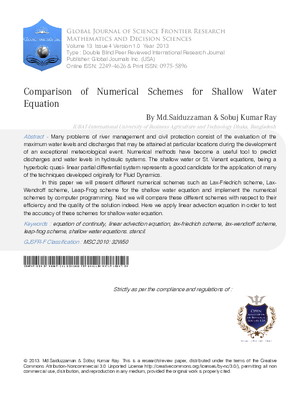### Comparison of Numerical Schemes for Shallow Water Equation

Authors

• Sobuj Kumar Ray

• Md.Saiduzzaman

Keywords:

equation of continuity, linear advection equation, lax-friedrich scheme, lax-wendroff scheme, leap-frog scheme, shallow water equations stencil

Abstract

Many problems of river management and civil protection consist of the evaluation of the maximum water levels and discharges that may be attained at particular locations during the development of an exceptional meteorological event. Numerical methods have become a useful tool to predict discharges and water levels in hydraulic systems. The shallow water or St. Venant equations, being a hyperbolic quasi- linear partial differential system represents a good candidate for the application of many of the techniques developed originally for Fluid Dynamics. In this paper we will present different numerical schemes such as Lax-Friedrich scheme, Lax-Wendroff scheme, Leap-Frog scheme for the shallow water equation and implement the numerical schemes by computer programming. Next we will compare these different schemes with respect to their efficiency and the quality of the solution indeed. Here we apply linear advection equation in order to test the accuracy of these schemes for shallow water equation.

How to Cite

Sobuj Kumar Ray, & Md.Saiduzzaman. (2013). Comparison of Numerical Schemes for Shallow Water Equation. Global Journal of Science Frontier Research, 13(F4), 29–46. Retrieved from https://journalofscience.org/index.php/GJSFR/article/view/854Published

2013-03-15

Issue

Section

Articles